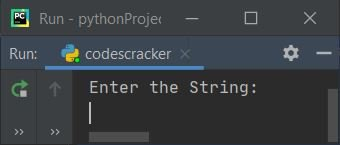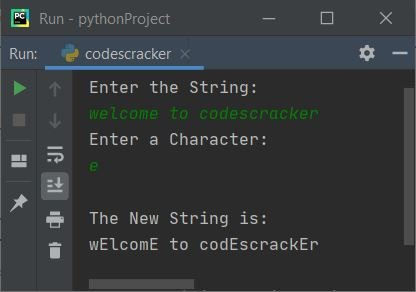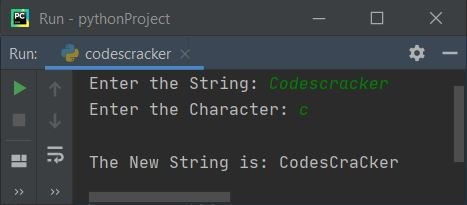# Python Program to Capitalize a Specific Character in a String

In this article, you will learn and get code in Python to capitalize any specific character (entered by the user) in a string (entered by the user).

For example, if the given string is codescracker, then the string after capitalizing the character, say c becomes CodesCraCker.

## Capitalize specific characters in strings

This program receives a string and a character from the user to capitalize the given character in the given string, as shown in the program given below:

```print("Enter the String:")
text = str(input())
print("Enter a Character:")
char = input()
textLen = len(text)
for i in range(textLen):
if char==text[i]:
if text[i]>='a' and text[i]<='z':
ascVal = ord(text[i])
ascVal = ascVal-32
ascVal = chr(ascVal)
index = i
text = text[:index] + ascVal + text[index+1:]
print("\nThe New String is:")
print(text)```

Here is its sample run:Now supply any string, say welcome to codescracker and a character, say e to capitalize all e in the given string as shown in the snapshot given below:The dry run of the above program with the same user input as provided in the sample run goes like this:

• Initial values: text = welcome to codescracker (entered by the user), and char = e (also entered by the user).
• The entered string is stored in text in such a way that:
• text=w
• text=e
• text=l
• and so on up to
• text=r
• Using len(), the length of text gets initialized to textLen. So textLen=23
• Now the execution of the for loop begins. Since range() contains only one value, the value of i stars with 0.
• Because 0 is less than textLen, program flow goes inside the loop, and the condition (of if) char==text[i] or e==text or e==w evaluates to be false, program flow does not go inside the if's body, rather the value of i gets incremented by 1. So i=1
• Again, the value of i is less than textLen; therefore, program flow again goes inside the loop and evaluates the condition of if.
• In this way, the execution continues until the value of i becomes equal to textLen.
• When the condition of if evaluates to be true, then we've checked whether the character at the current index of text[] is greater than or equal to a and less than or equal to z or not.
• If this condition is satisfied, then we only have to capitalize the character. Otherwise, continue to check for the next character. Because only alphabets (a–z) can be capitalized.
• The ord returns the Unicode (ASCII value) of a character. And chr() returns the character corresponding to its Unicode.

Note: The text[:2] referred to elements from the starting (0th) to the second (1st) index. Whereas text[2:] referred to elements from the second (2nd) index to the last.

You can also replace the following block of code:

```if char==text[i]:
if text[i]>='a' and text[i]<='z':```

with

```if text[i]>='a' and text[i]<='z':
if char==text[i]:```

#### Modified version of the previous program

This is a modified version of the previous program. The end is used to skip the printing of the automatic newline.

```print(end="Enter the String: ")
text = str(input())
print(end="Enter the Character: ")
char = input()
if char>='A' and char<='Z':
ascVal = ord(char)
ascVal = ascVal + 32
ascVal = chr(ascVal)
char = ascVal
textLen = len(text)
for i in range(textLen):
if char==text[i]:
if text[i]>='a' and text[i]<='z':
ascVal = ord(text[i])
ascVal = ascVal-32
ascVal = chr(ascVal)
index = i
text = text[:index] + ascVal + text[index+1:]
print("\nThe New String is: " + text)```

Here is its sample run with user input: CodescrackerPython Online Test

« Previous Program Next Program »